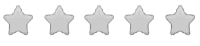See inside this book

# Math-U-See Gamma Student Pack

\$42.00
No tax
Demme Learning
Math
3
P-MA03-62Leave a Review

#### Description

New! Math-U-See Gamma - Student Pack

Math-U-See is a complete, skill-based, multi-sensory homeschool math curriculum for grades K-12 that?s designed to teach students skills in a logical sequence.

The Gamma Student Pack is for those who already have the other required components for the course. It contains the Student Workbook with lesson-by-lesson worksheets, systematic review pages, and Application & Enrichment pages as well as Gamma Tests.

Once students have mastered the concepts of addition and subtraction (covered in Alpha and Beta), they are ready for multiplication. Gamma teaches single-digit facts and multiple-digit multiplication skills.

Major Concepts and Skills Include:

Using strategies based on place value and properties of operations to multiply Fluently multiplying any combination of whole numbers Solving for an unknown factor Solving abstract and real-world problems involving addition, subtraction, and multiplication Measuring and computing area Relating concepts of area to addition and multiplication

Skip counting as a precursor to multiplication Adding and subtracting time in hours and minutes Multiplying, adding, and subtracting U.S. currency and standard units of measure Representing, recording, and interpreting data Understanding of basic fractions Estimating and solving measurement problems